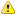﻿ Reports > Statistics > Performance

Performance

The view is docked into a Report Page. For more information see the Report Page chapter.

The Performance view has three separated parts:

Performance Summary based on total equity and cash transactions

This summary uses the total equity list for its calculations. Because of this only the date filters are applied in this section.

Columns:

Starting Total Equity (date) - the first available total equity value after the starting date set in the filter

Ending Total Equity (date)  - the last available total equity value before the end date set in the filter

Net Profit - the difference between the Starting Total Equity and the Ending Total Equity

Net Profit % - the net profit percentage of the Starting Total Equity

Annualized Gain - Annual gains between the Starting Total Equity and the Ending Total Equity

Interest - interest summary. Paid and received.

Other fees - other fees charged like broker fee, real time data fee etc.Note. This section is only available if there are enough elements in the Total Equity list. For more information please see the Trading Menu Generate Total Equity section and the Equity view.

Performance Summary based on positions

This summary uses the positions for its calculations. All filters are applied.

Columns:

Realized Profit: the summary of all closed positions profit.

Realized Profit %: the percentage of the realized profit to the Starting Total Equity.

Commission: the summary of all closed positions commission.

Performance detailed

The Performance Detailed section is a matrix view.

There are three columns defined:

Total - values for both long and short positions

Long - values only for long positions

Short - values only for short positions

Rows

Number of positions - shows the number of the positions for each column

Average Profit & Loss - shows the average profit or loss for each column. Calculation: sums the positions P&L and divides it with the number of positions

Longest position holding time - shows the longest time of a position's starting and end date.

Average position holding time - shows the average position holding time value. Calculation: sums the positions holding times and divides it with the number of positions

Expectation - mathematical expectation in base currency

Expectation % - mathematical expectation in percent

Profit Factor -  is the ratio in percentage between the the sum of all the win trades (Gross profit) and the sum of all the loss trades (Gross loss)

Average Initial Risk - shows the average initial risk

Average Initial Risk % - shows the average initial risk in percent

Winning positions - shows the number of those positions which are profitable

Winning % - shows the percentage of the winning positions. Calculation: number of Winning positions/ (number of Winning positions + number of Losing positions)

Gross profit - shows the P&L sums for the winning positions

Average profit - shows the average profit for winning positions. Calculation: sums the Winning profits and divides it with the number of Winning positions

Max consecutive - shows the number of max consecutive wins.

Average R Multiple - average R Multiple of winning positions.

Losing positions - shows the number of those positions which are losing

Losing % - shows the percentage of the losing positions.  Calculation: number of losing positions / number of winning positions + number of losing positions

Gross loss - shows the P&L sums for the losing positions

Average loss - shows the average loss for losing positions. Calculation: sums the loss and divides it with the number of Losing positions

Max consecutive - shows the number of max consecutive loses.

Average R Multiple - average R Multiple of losing positions.Note. Average R Multiple, Average Initial Risk and Average Initial Risk % values calculated only for those positions where the stop is given.